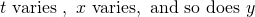# Student’s Question #2This question is with regards to parametric equations. We are givenand my student have difficulties finding the equation of the line of symmetry.

So let us first look at how parametric equations actually work here, since most students are really confused with it. Parametric equations are a set of equations that is “linked” or related using a parameter which is a variable. In this case, bothare connected using a new parameterhere. And as.

Considering that we found the cartesian equation in (i),, observe that ifthen, so for every x-value, we can map to two y-value, the positive and negative one. Thus the line of symmetry will be the x-axis here.

Not readable? Change text.